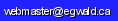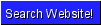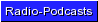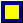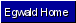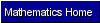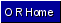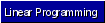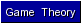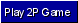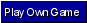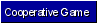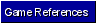Operations Research - Game Theory

by

Elmer G. Wiens

Egwald's popular web pages are provided without cost to users.
Please show your support by joining Egwald Web Services as a Facebook Fan:Follow Elmer Wiens on Twitter:Game Theory - Introduction | Battle of the Sexes | Prisoner's Dilemma | Free Rider | Game of Chicken | Play a Game Online | Metagame Example

Game Theory - Introduction

Game theory studies situations in which parties compete, and also possibly cooperate, to influence the outcome of the parties' interaction to each party's advantage. The situation involves conflict between the participants — called players — because some outcomes favour one player at the expense of the other players. What each player obtains from a particular outcome is called the player's pay-off. Each player can choose among a number of actions to influence his pay-off. However, each player's pay-off depends on the other players' choices. Moreover, outcomes can also be influenced by "chance occurences." (see the Wikipedia's introduction to game theory.)

A two-person game has two players. A game in which one player wins what the other player loses is called a zero-sum game. The theory of two-person zero-sum games is the foundation of more complicated games, such as games with more than two players (n-person games), and games in which the players can benefit through cooperation, with or without collusion, side payments, or binding agreements. This introduction is primarily concerned with two-person zero-sum games.

The irreconcilable, conflicting interests between the two players in a zero-sum game resemble parlour games and military encounters between enemy states. Players make moves and counter-moves, until the rules of engagement declare the game is ended. The rules of engagement determine what each player can or must do at each stage (the available and/or required moves given the circumstances of the game at this stage) as the game unfolds. For example, in the game rock, paper, scissors both players simultaneously make one move, with rock beating scissors beating paper beating rock. While this game consists of only one move, games like chess require many moves to resolve the conflict.

A player's strategy is defined as a plan of action that determines the player's move at each stage of the game (depending on the circumstances of the game at each stage), from the player's first move all the way to the player's final move. Different ploys for play produce different strategies. A player's tactics specify a player's "line of attack/defense" at a particular stage of the game. Different tactics yield different strategies. Suppose, for example, players agree to play "ten games" of rock, paper, scissors, a game of ten moves. One strategy is the tactic of selecting rock, paper, or scissors with probability 1/3 at each of the ten stages. In rapid play, however, choosing moves at random is difficult. Consequently, detectable patterns of play could emerge. Another strategy is the tactic of replicating the opposing player's previous move in the 3rd, 7th, and 10th stage (tit-for-tat tactics), and "going with intuition" at the other stages. An alternative strategy is the exhaustive strategy whereby each player's move is determined, at a given stage, on the network of all possible moves both players made in the previous stages. Whether exhaustive strategies are actually available depends on such factors as the player's memory and the rate at which the game is played.

Thus, a player's strategy provides him with a move depending on what the other player has done, and/or chance events, as the game unfolds. Different moves and/or means to choose the move in a given situation are embedded in different strategies. A game with a finite number of stages and a finite number of moves at each stage is called a finite game. A finite game has a finite number of distinct strategies available to each player. The game of chess is a finite game, despite its complexity and vast number of strategies.

The choice of a particular strategy by each player determines the pay-off to each player.

Colonel Blotto Attack and Defense Game

The following game of strategy illustrates the concepts introduced above.

Colonel Blotto must defend two cities with one indivisible regiment of soldiers. His enemy, Colonel Sotto, plans to attack one city with his indivisible regiment. City I has a value of 10 units, while City II has a value of 5 units. If Colonel Sotto attacks a defended city, Sotto loses the battle and obtains nothing. If Colonel Sotto attacks an undefended city, he obtains the value of the city, while Colonel Blotto loses the value of the city. Neither Colonel knows what the other plans to do.

Normal Form.

The following tableau summarizes this situation of conflict — a two-person, zero-sum game. This representation of the game is called the normal form. It lists Sotto's strategies vertically, and Blotto's strategies horizontally. The numbers represent the gains to Sotto and the losses to Blotto, for each choice of strategy.

 Attack and Defense Tableau Colonel Blotto Defend City ID1 Defend City IID2 Colonel Sotto Attack City IA1 0 10 Attack City IIA2 5 0

Colonel Sotto has two "pure strategies," Attack City I and Attack City II; Colonel Blotto has two "pure strategies," Defend City I and Defend City II. Each Colonel weighs each pure strategy against his enemy's pure strategies.

Examining his options, Colonel Sotto thinks, "Blotto might defend City I, since City I is worth more than City II. Why don't I attack City II"? Conversely, Colonel Blotto thinks, "Sotto might attack City II, since he thinks I will defend City I. Why don't I defend City II"? Thinking further into the game, Sotto thinks, "If Blotto is trying to read my mind, he might defend City II. Maybe I should attack City I after all."

Each Colonel's attempt to read his enemy's mind degenerates into an infinite regression.

However, a solution concept lurks in the Colonels' "gedank" experiments. Sotto attacks each city with positive probability, while Blotto defends each city with positive probability. Furthermore, since City I is worth twice as much as City II, the probability that Blotto defends City I should be twice as great as the probability that he defends City II. Conversely, since Sotto loses in a head-to-head confrontation with Blotto, the probability that Sotto attacks City II should be twice as great as the probability that he attacks City I. Both probabilities for each Colonel must add-up to one. Therefore, the optimal strategy for Colonel Blotto is to defend City I with probability 2/3, and to defend City II with probability 1/3. In the meantime, the optimal strategy for Colonel Sotto is to attack City I with probability 1/3, and to attack City II with probability 2/3.

Strategies consisting of probability weighted pure strategies are called mixed strategies.

If Colonel Sotto plays the mixed strategy (Attack City I, Attack City II) = (1/3, 2/3), he expects to gain:

0 * 1/3 + 5 * 2/3 = 3 1/3 units if Blotto plays Defend City I,

10 * 1/3 + 0 * 2/3 = 3 1/3 units if Blotto plays Defend City II.

If Colonel Blotto plays the mixed strategy (Defend City I, Defend City II) = (2/3, 1/3), he expects to lose:

0 * 2/3 + 10 * 1/3 = 3 1/3 units if Sotto plays Attack City I,

5 * 1/3 + 0 * 2/3 = 3 1/3 units if Sotto plays Attack City II.

Colonel Sotto's strategy assures him of an expected gain of at least 3 1/3 units; Colonel Blotto's strategy assures him of an expected loss of not more than 3 1/3 units. The value of the game is 3 1/3 units.

Extensive Form.

The next diagram shows the extensive form, or game tree, for the Colonel Blotto Game. The blue circle represents Colonel Sotto's decision node, while the red circles represent Colonel Blotto's decision nodes. Colonel Blotto's decision nodes are contained in the yellow ellipse, because Blotto does not know which node he actually is in, since Blotto and Sotto choose their strategies simultaneously. The numbers at the green nodes are the pay-offs to (Sotto, Blotto) with the strategies chosen.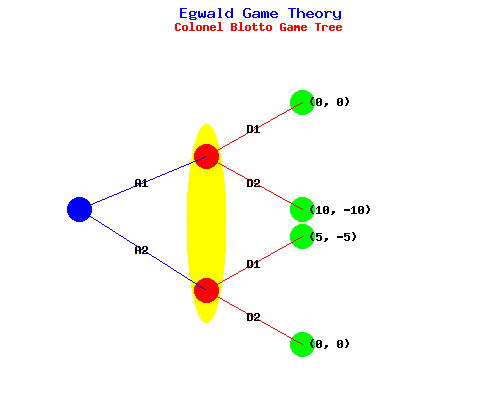The yellow ellipse is called the information set for Colonel Blotto, since Blotto just knows that he is at one or the other node in the ellipse.

The game is symmetrical in the sense that I can start the game tree with Colonel Blotto's decision node instead.

"Doc" Holliday's Revenge

On October 26, 1881, the bad blood between the Earps, Clantons, and McLaurys came to a head at the OK Corral. Billy Clanton, Frank McLaury, and Tom McLaury were killed. Doc Holliday, Virgil and Morgan Earp were injured. Miraculously, Wyatt Earp was unharmed, while the unarmed Isaac "Ike" Clanton survived by running away. Many people believed that Doc Holliday shot-gunned an unarmed Tom McLaury in the back as he was attempting to flee the scene.

Ike Clanton and his friends and associates, known as the "cowboys," swore to get their revenge on the Earps and Holliday. In the ensuing months, Morgan Earp was murdered and Virgil Earp seriously wounded in an ambush. A few days later, Wyatt Earp apparently shot and killed Frank Stillwell, a Clanton associate, and another man believed involved in the ambush. Over the next few years, many more of the "cowboys" were killed.

Although close to death from tuberculosis, in 1887 Doc Holliday decides to look up Ike Clanton and to settle their differences once and for all. On June 1, 1887, Doc Holliday and J.V. Brighton corner Ike Clanton near Springerville, Arizona. Doc tells J.V. to stay out of it for the time being.

Ike and Doc have each a pistol and shotgun. Ike and Doc, spurnning their pistols in favour of their shotguns, press forward towards each other. At long range, Ike — with his cowboy background — is a better shot than Doc. At middle range, Doc — the seasoned gunfighter — out-guns Ike. Both desperadoes are deadly at close range. The probabilities that either rogue will kill the other with a blast from his single-shot shotgun appear in the following table:

Kill Probability
Long RangeMiddle RangeClose Range
Ike Clanton0.50.61.0
Doc Holliday0.30.81.0

The pay-off to Doc is 10 units if Doc survives and Ike is killed, -10 units if Doc is killed and Ike survives. Doc and Ike's strategies are:

Lblast at long range
Mif enemy has not shot, blast at middle range; otherwise, blast at close range
Cblast enemy at close range

Normal Form.

Always the consummate gambler, Doc Holliday correctly computes his pay-off matrix as:

Shoot-out
Pay-off
Ike's Strategies
ILIMIC
Doc's
Strategies
DL-2-7-7
DM026
DC0-20

Furthermore, Doc realizes that strategy DM dominates strategies DL and DC, in that his expected pay-off is higher with DM no matter what Ike does. If Ike is rational enough — if Ike correctly computes his pay-off matrix as the negative of Doc's pay-off matrix — Ike will choose strategy IL, because IL dominates strategies IM and IC provided Doc chooses strategy DM.

The pair of pure strategies, the dominant strategies (DM, IL), is the "solution" to the game. The value of the game is 0, the pay-off when Doc chooses strategy DM and Ike chooses IL.

The pair of strategies, (DM, IL), determines a saddle point of the pay-off matrix. Notice that the pay-off matrix entry, "0," is the largest entry in the IL column and the smallest entry in the DM row. A strategy pair whose pay-off matrix entry is a saddle point is called an equilibrium strategy pair.

Extensive Form.

The next diagram shows the extensive form, or game tree, for Doc Holliday's Game. The blue circle represents Doc Holliday's decision node, while the red circles represent Ike Clanton's decision nodes. Ike Clanton's decision nodes are contained in the yellow ellipse, because Ike does not know which node he actually is in, since Ike and Doc choose their strategies simultaneously. The numbers at the green nodes are the pay-offs to (Doc, Ike) with the strategies chosen.The yellow ellipse is called the information set for Ike Clanton, since Ike just knows that he is at one of the three nodes in the ellipse.

The game is symmetrical in the sense that I can start the game tree with Ike Clanton's decision node instead.

Doc Holliday survived the show-down, apparently dying from tuberculosis on November 8, 1887 in Colorado. Not so funny, after all.

Graphical Solution of a 2 x n or m x 2 Game

A two-person zero-sum game with a pay-off matrix with dimensions either 2 x n, or m x 2 can be solved graphically. For example, consider the game with the 2 by 4 pay-off matrix:

Pay-Off
Matrix
Player II's Strategies
II1II2II3II3
Player I's
Strategies
I14313
I20521

Since this matrix does not have a saddle point, one must resort to mixed strategies for a solution. If Player I plays strategy I1 with probability ß, and strategy I2 with probability (1 - ß) his expected returns, R, against each of Player II's strategies are:

II's strategyPlayer I's Return
II14 * ß + 0 * (1 - ß) = 0 + 4 * ß = R1
II23 * ß + 5 * (1 - ß) = 5 - 2 * ß = R2
II31 * ß + 2 * (1 - ß) = 2 - 1 * ß = R3
II43 * ß + 1 * (1 - ß) = 1 + 2 * ß = R4

Player I's expected return against a particular strategy of Player II is a function of ß, a straight line. The next diagram graphs these straight-line functions as ß varies from 0 to 1. The probability ß is graphed along the x-axis, while Player I's return Ri against Player II's ith strategy is graphed along the y-axis.Player I's "Optimal" Strategy.

If Player I sets ß = .4, Player I can expect at least a return R = 1.6 against any strategy or combination of strategies that Player II chooses to play. The (ß, R) = (0.4, 1.6) point occurs at the intersection of the R1 and R3 lines.

Player II's "Optimal" Strategy.

Since Player's I's mixed strategy (0.4, 0.6) assures him of an expected gain of 1.6 units, Player II wants a strategy that will limit his expected loss to 1.6 units. Moreover, Player II can restrict his choice of strategies to II1 and II3, because the diagram shows that strategies II2 and II4 expose him to losses greater than 1.6 units.

If Player II plays strategy II1 with probability µ, and strategy II3 with probability (1 - µ) his expected losses L against Player I's mixed strategies are:

 L = R1 * µ + R3 * (1 - µ),                   L = (4 * ß) * µ + (2 - ß) * (1 - µ), or                 L = (5 * µ - 1) * ß + (2 - 2 * µ)

If Player II sets µ = 1 / 5 = .2 , his expected losses are:

 L = (5 * (1/5) - 1) * ß + (2 - 2 * (1/5) ) = 8 / 5 = 1.6

Since Player II can limit his losses independently of Player I's choice of mixed strategy, Player II's mixed strategy (0.2, 0, 0.8, 0) guarantees him of an expected loss of no greater than L = 1.6.

Game Solution.

Player I plays a mixed stragey of   (0.4, 0.6)   and Player II plays a mixed strategy of   (0.2, 0, 0.8, 0).  The value of the game is 1.6 units.

The Min-Max Theorem for Matrix Games

A matrix game can be described by its pay-off matrix, an m by n matrix A  =  [ai, j], the set X of all probability distributions x on the integers {1, 2, . . . , m}, and the set Y of all probability distributions y on the intergers {1, 2, . . . , n}. Thus

 xT = (x1, x2, ... , xm), with x1 + x2 + ... + xm = 1 yT = (y1, y2, ... , yn), with y1 + y2 + ... + yn = 1 A = a1,1 a1,2     . . . . .    a1,n-1 a1,n a2,1 a2,2     . . . . .    a2,n-1 a2,n               . . . . . . . . . . .           am,1 am,2     . . .     am,n-1 am,n

If Player I selects a probability distribution x and Player II selects a probability distribution y, the pay-off to Player I is P(x, y) while the pay-off to Player II is -P(x, y), where:

 P(x, y) = xT * A * y

ie, the expected pay-off equals the product of the row vector xT and the matrix A, (another row vector), and the column vector y

Lower Value: Max-Min Game.

If Player I announes that he will use a particular strategy x in X, Player II should choose the strategy y0 in Y that minimizes the expected pay-off to Player I. That is, Player II should search for that y0 where:

P(x, y0) = miny in Y P(x, y)

Therefore, Player I should choose x0 in X so that:

vI = miny in Y P(x0, y) = maxx in X [ miny in Y P(x, y) ]

The number vI is called the lower value, or the maxmin value, of the game.

Upper Value: Min-Max Game.

If Player II announes that he will use a particular strategy y in Y, Player I should choose the strategy x0 in X that maximizes the expected pay-off to Player I. That is, Player I should search for that x0 where:

P(x0, y) = maxx in X P(x, y)

Therefore, Player II should choose y0 in Y so that:

vII = maxx in X P(x, y0) = miny in Y [ maxx in X P(x, y) ]

The number vII is called the upper value, or the minmax value, of the game.

Min-Max Theorem for Matrix Games.

If x0 and y0 are the strategies chosen as indicated above, then:

vI = vII = v = the value of the game = P(x0, y0)

If x is any mixed strategy in X, and y is any mixed strategy in Y, then:

P(x, y0) <= P(x0, y0) <= P(x0, y)

Thus the strategy pair (x0, y0) is the solution to the game. The two strategies provide a saddle point in mixed strategies in the Cartesian product domain of X x Y.

Linear Programming Solution for Matrix Games

One can conveniently illlustrate the method used to turn a matrix game into a linear programming problem with an example (Hadley, 427). Suppose that player I has 3 strategies, player II has 5 strategies, and the payoff matrix A is given by:

P
l
a
y
e
r

I
P l a y e r   II
 3 5 0 9 6 2 6 8 1 2 1 7 4 9 3
= A

If player I plays strategy 2, his payoff depends on which strategy player II has chosen to play. If player II knows player I is playing strategy 2, she should play her 4th strategy, because then she only pays him 1 unit. But if player I plays strategy 1 instead, she must pay him 9 units. I assume that players know the payoff matrix A, but that players 'announce' their strategies simultaneously. Player I wants to maximize the payoff; player II wants to minimize the payoff.

The Min-Max Theorem suggests each player determine the choice of strategies on the basis of a probability distribution over the player's list of strategies. That is, player I chooses row 1, 2, or 3 with probabilities x1, x2,and x3. Player II chooses the columns with probabilities y1, y2, y3, y4, and y5. Note that the x's and y's must be non-negative and each set must add to one.

To turn this into a linear programming problem write:

Primal Linear Program

Maximize P = x4, with x4 nonnegative
 3*x1 + 2*x2 + 1*x3 - 1*x4 >= 0 5*x1 + 6*x2 + 7*x3 - 1*x4 >= 0 0*x1 + 8*x2 + 4*x3 - 1*x4 >= 0 9*x1 + 1*x2 + 9*x3 - 1*x4 >= 0 6*x1 + 2*x2 + 3*x3 - 1*x4 >= 0 1*x1 + 1*x2 + 1*x3 + 0*x4 = 1

where the extra variable, x4, is the expected payoff (the value) of the game.

The equation,

Maximize P = x4, with x4 nonnegative,

is the objective function. Player I wants to maximize the objective function, the expected payoff.

Then there is an equation for each column of A. Why? If player II plays her 1st strategy, the expected payoff to player I is:

3*x1 + 2*x2 + 1*x3.

If player I choses his probability distribution optimally, his expected payoff should be greater than or equal x4, the expected value of the game. This argument applies to each of A's columns.

The dual linear program to the primal linear program is:

Dual Linear Program

Minimize D = y6, with y6 nonnegative
 3*y1 + 5*y2 + 0*y3 + 9*y4 + 6*y5 - 1*y6 <= 0 2*y1 + 6*y2 + 8*y3 + 1*y4 + 2*y5 - 1*y6 <= 0 1*y1 + 7*y2 + 4*y3 + 9*y4 + 3*y5 - 1*y6 <= 0 1*y1 + 1*y2 + 1*y3 + 1*y4 + 1*y5 + 0*y6 = 1

The equation,

Minimize D = y6, with y6 nonnegative,

is the objective function. Player II wants to minimize the objective function, the expected payoff.

Then there is an equation for each row of A. Why? If player I plays his 1st strategy, the expected payoff to player II is:

3*y1 + 5*y2 + 0*y3 + 9*y4 + 6*y5.

If player II choses her probability distribution optimally, her expected payoff should be less than or equal y6, the expected value of the game. This argument applies to each of A's rows.

If one solves this linear programming problem using one of the available methods, the optimal progams:

xT = (x1, x2, x3) = (0.667, 0.333, 0) and

yT = (y1, y2, y3, y4, y5) = (0.889, 0, 0.111, 0, 0),

are the Max-Min and Min-Max solutions, while the value, x4 = y6 = 2.667, is the value of the matrix game.

Value of the Game - Nonnegative.

To ensure that the value of an arbitrary matrix game is nonnegative when using linear programming to find its solution, add the absolute value of the most negative entry of the pay-off matrix to each entry of the pay-off matrix. After solving the modified game, subtract this constant from its value to get the value of the original matrix game.

You can play this matrix game using the form below.
You are the row player, Player I.

Use the bonus random number to select the row you want to play.
Can you can beat the value of the game over a sequence of games?

    y1 y2 y3 y4 Click the row you want to play. The program selects a column using its optimal strategy. It ignores the row you choose. x1 3 5 0 9 6 x2 2 6 8 1 2 x3 1 7 4 9 3

Optimal Strategies
x1 = 0.667 x2 = 0.333 x3 = 0
y1 = 0.889 y2 = 0 y3 = 0.111 y4 = 0 y5 = 0
The value of the game = 2.667
Your bonus random number (0-100) is 29

Create and play your own matrix game! Specify the payoff matrix dimensions and
then input the payoff matrix entries on the webpage at: Play Own Game.

Properties of Optimal Mixed Strategies.

Suppose Player I plays his optimal strategy , xT = (x1, x2, x3) = (0.667, 0.333, 0), and Player II plays one of her pure strategies. The vector xT * A shows the amount Player II can expect to pay to Player I for each pure strategy she plays.

xT * A = (2/3, 1/3, 0) *
 3 5 0 9 6 2 6 8 1 2 1 7 4 9 3
= (2.6667 5.3333 2.6667 6.3333 4.6667)

Notice that only pure strategies 1 and 3 ensure Player II of paying the minimum of 2.6667 units, when Player I plays his optimal mixed strategy.

Likewise, the vector A * y shows the amount Player I can expect to receive from Player II for each pure strategy he plays against her optimal strategy.

A * y =
 3 5 0 9 6 2 6 8 1 2 1 7 4 9 3
* (0.88888889, 0, 0.11111111, 0, 0)T = = (2.6667, 2.6667, 1.3333)T

Notice that only pure strategies 1 and 2 ensure Player I of receiving the maximum of 2.6667 units, when Player II plays her optimal mixed strategy.

Worthwhile Strategies.

Pure strategies that appear in an optimal strategy with positive probability are called worthwhile strategies (Thomas, 37).

Set of Optimal Mixed Strategies.

For each player in a two-person zero-sum matrix game, the set of optimal mixed strategies is a closed, convex set (Karlin, 36).

For each player, the optimal strategy calculated from the equivalent linear programming problem is an extreme point of the player's set of optimal strategies. This follows because in a linear programming problem, an optimal program obtains at a vertex of the feasible set, and the feasible set must contain the set of optimal strategies.

Pure Strategy as a Mixed Strategy.

A pure strategy is a mixed strategy with the component corresponding to the probability of playing the pure strategy equal to 1.

The Snow-Shapley Theorem for Matrix Games.

Consider again the two-person zero-sum game with pay-off matrix:

A =
 3 5 0 9 6 2 6 8 1 2 1 7 4 9 3

This game has optimal mixed strategies:

xT = (x1, x2, x3) = (0.667, 0.333, 0) and

yT = (y1, y2, y3, y4, y5) = (0.889, 0, 0.111, 0, 0),

If one strikes out the rows and columns of A corresponding to strategies played with zero probability, one gets the reduced matrix M corresponding to the reduced matrix game with pay-off matrix M:

M =
 3 0 2 8

This procedure of striking out "worthless strategies" produces a matrix M whose inverse M(-1) exists:

M(-1) =
 0.3333 0 -0.0833 0.125

Furthermore, letting eT = (1, 1), ie the vector whose components are all one, the value of the game, v, is given by:

v = eT * M(-1) * e = 2.6667.

Moreover, the optimal strategies for Player I and Player II in the reduced game are:

x0 = v * eT * M(-1) = (0.6667, 0.3333), and

y0 = v * M(-1) * e = (0.8889, 0.1111).

Finally, the value of the original game is also v, and the optimal strategies for Player I and Player II in the original game are obtained by setting to zero the probability of playing a worthless strategy.

Snow-Shapley Theorem.

Consider any two-person zero-sum matrix game with pay-off matrix A. If x is an extreme point of X0, Player I's set of optimal strategies, and if y is an extreme point of Y0, Player II's set of optimal strategies, there exists a square, nonsingular submatrix M of A such that:

v = eT * M(-1) * e,

x0 = v * eT * M(-1),

y0 = v * M(-1) * e,

where e is the vector of the same dimension as M all of whose components equal 1, v is the value of the game, and x0 and y0 correspond to the non-zero components of x and y, respectively.

References

• Black, Max. Perplexities. Ithaca: Cornell, 1990.
• Brahms, Steven J. Biblical Games: A Strategic Analysis of Stories in the Old Testament. Cambridge, Mass: MIT Press, 1980.
• Brahms, Steven J. Theory of Moves. Cambridge: Cambridge UP, 1994.
• Dresher, Melvin. Games of Strategy: Theory and Applications. Englewood Cliffs: Prentice-Hall, 1961.Copyright © Elmer G. Wiens:   Egwald Web ServicesAll Rights Reserved.    Inquiries